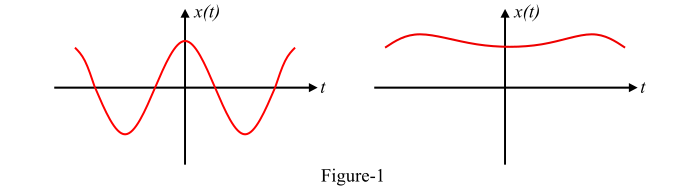# Signals and Systems ŌĆō Classification of Signals

## What is a Signal?

A signal can be defined in one of the following ways −

• Anything that conveys information can be termed as a signal.

• A signal can also be defined as a single valued function of one or more independent variables which has some information.

• A signal may also be defined as any physical quantity that varies with time or any other independent variable.

A signal may be represented in time domain or frequency domain. Some common examples of a signal are human speech, electric current, electric voltage, etc.

By the definition, a signal can be a function of one or more independent variables such as time, position, pressure, temperature, etc. If a signal is a function of only one independent variable, it is called one-dimensional signal and if a signal is a function of two independent variables, it is called two-dimensional signal.

## Classification of Signals

Depending upon the nature and characteristics in the time domain, the signals may be classified into two categories −## Continuous Time Signals

The signals which are defined for every instant of time are called as continuous time signals. The continuous-time signals are also called as analog signals. In case of continuous time signals, the independent variable is time. The graphical representation of continuous-time signals is shown in Figure-1.## Discrete Time Signals

Those signals which are defined only at discrete instants of time are called as discrete time signals. The amplitude of discrete time signals is continuous but these signals are discrete in time. The amplitude of a discrete time signal between two time instants is just not defined. For the discrete time signals, the independent variable is time, denoted by n. As these signals are defined only at discrete time instants, therefore, they are given by a sequence x(n) or x(nT) where, n is an integer.

The graphical representation of discrete time signals is shown in Figure-2.### Both discrete-time and continuous-time signals may be further classified as follows −

• Deterministic Signals – A deterministic signal is the one that exhibits no uncertainty of amplitude and phase at any instant of time. These signals have a regular pattern. Sine wave, exponential signals, square wave, etc. are the examples of deterministic signals.

• Random Signals – A signal that has uncertainty about its occurrence is known as random signal. A random signal has irregular pattern and cannot be represented by the mathematical equations. Thermal noise generated in an electric circuit is a common example of random signal.

• Periodic Signals – A periodic signal is defined as a signal which has a definite pattern which repeats itself at regular intervals of time.

• Aperiodic Signals – A signal which does not repeat at regular intervals of time is known as aperiodic signal. The aperiodic signals are also called the non-periodic signals.

• Energy Signals – A signal is said to be an energy signal if and only if its total energy is finite. The average power of an energy signal is zero, i.e., P = 0. Examples of energy signals are aperiodic signals.

• Power Signals – If a signal has finite average power (i. e. , 0 < ­Øæā < ∞), it is called a power signal. The total energy of a power signal is zero (i.e., E = 0). Periodic signals are the examples of power signals.

• Causal Signals – A continuous-time signal x(t) is said to be causal signal if x(t) = 0 for t < 0. Similarly, a discrete time signal x(n) is said to be causal signal if x(n) = 0 for n < 0. Thus, a causal signal does not exist for negative time. Example: u(t) is a causal signal.

• Anti-Causal Signals – A continuous-time signal x(t) is said to be anticausal signal if x(t) = 0 for t > 0. Similarly, a discrete-time signal x(n) is said to be anti-causal signal if x(n) = 0 for n > 0. Therefore, an anticausal signal does not exist for positive time. Example: u(-t) is an anticausal signal.

• Non-Causal Signals – Those continuous-time or discrete-time signals which are not causal, are known as non-causal signals. For example, a signal which exists in positive as well as negative time neither causal nor anti-causal but it is a non-causal signal.

• Even Signals – Those signals which are symmetrical about the vertical axis or time origin are known as even signals. They are also known as symmetrical signals. Cosine wave is an example of even signal.

• Odd Signals – The signals which are anti-symmetrical about the vertical axis are said to be odd signals. The odd signals are also known as anti-symmetrical signals. Sine wave is an example of an odd signal.